### Initial calculations

In subroutine initialcfdfem.f the following fields are calculated:

• For each node i in the fluid, the distance from this node to the nearest solid surface node. This distance is stored in field yy(i) and the corresponding nearest surface node in jyy(i). They are needed for the turbulence model.
• For each solid surface node, the distance to the nearest in-flow node. It is stored in field xsolidsurf. This quantity is also needed for the turbulence model.
• For each node i the adjacent element height dh(i). This is the minimum of the height of all elements to which the node belongs. The height of an element j is its volume divided by the largest facial area times a factor (e.g. 1 for a hexahedral element and 3 tetrahedral element). This height is stored in field dhel(j) and is used to determine dh(i). From dh(i) the local time increment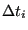is calculated.
• For shallow water calculations: the depth in all fluid nodes (this is the element length in the direction of the gravity vector).
• Initial conditions for the turbulence parameters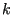and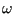.
• The value of the conservative variables in all fluid nodes starting from the physical variables. The conservative variables, stored in field vcon(1..nk,0..mi(2)), are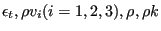and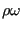. For efficiency first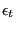is stored for all nodes, then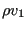and so on..., since they are solved for separately (so a single pointer suffices to switch between the fields). The physical variables are the static temperature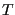, the velocity components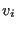, the static pressue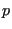and the turbulence parametersand. They are stored in field vold(0..mi(2),1..nk) in the way conventional to structural calculations, i.e. first all parameters for node 1, then for node 2....

The fields calculated in initialcfdfem frequently contain distances between nodes, which may have changed since the last call to compfluid.